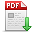叶继华,郭祺玥,江爱文,等.基于特征子空间直和的跨年龄人脸识别方法[J].郑州大学学报(工学版),2021,42(05):7-12. 点击复制 基于特征子空间直和的跨年龄人脸识别方法() 分享到： var jiathis_config = { data_track_clickback: true };

42

2021年05期

7-12

2021-09-10

文章信息/Info

Title:
Cross-age Face Recognition Method ba＜x＞sed on Feature Subspace Direct Sum

A

Abstract:
The present methods ignore the correlation between age factor and identity factor to some extent. Therefore, we introduce the direct sum module on the basis of the multi-task convolutional neural network that simultaneously performs two tasks of face recognition and age classification, and propose the feature subspace with direct sum multi-task convolutional neural network (FSDS-CNN). The network first extracts the facial deep feature from the input face image through a convolution unit stacked by multiple convolution la＜x＞yers, batch normalization la＜x＞yers, activation function la＜x＞yers and pooling la＜x＞yers, and then two parallel discriminative subnets, namely the identity subnet and the auxiliary age subnet, extract the identity-related feature and age-related feature from the deep feature shared by the convolution unit to pass to the corresponding discriminator for multi-task classification. At the same time, by leveraging the direct sum module proposed in our paper, the direct sum constraint is applied to the feature subspaces corresponding to these two related features, then the redundant information between the two feature subspaces is eliminated as much as possible, so that the correlation between identity-related feature and age-related feature is decreased as much as possible. Through the joint supervised learning of multiple loss functions, the network can effectively separate the age information from the face identity feature and obtain age-invariant face identity feature that is robust with age. We first conduct explore experiment for the setting of the two important hyperparameters (the number of eigenvectors and the weight of direct sum loss) of the direct sum module on the Morph Album 2 dataset, and finally determine that the number of eigenvectors is 25 and the weight of direct sum loss is 10. After that, we conduct cross-age face recognition and verification experiments on three public -domain benchmark aging datasets, and compare with 10 representative methods in recent years. In the Morph Album 2 dataset, our method achieves the result of 98.41% on the evaluation metric of Rank-1 Identification Rate ( higher is better), which is second along all of the methods in the CACD-VS dataset, our method achieves the result of 99.2% on the evaluation metric of Accuracy ( higher is better), which is the second, and the result on the evaluation metric of AUC (Area Under Curve, higher is better ) is 99.7%, which achieves an optimal value along all of the methods and increases by 0.1% compared with the second model in the Cross-Age LFW dataset, our method achieves the result of 10.1% on the evaluation metric of Equal Error Rate (EER, less is better), and achieves the result of 10.2% on the evaluation metric of the false non-match rate when false match rate is 0.1 (FNMR@FMR=0.1, less is better), all of which achieve the optimal value and decrease by 4.7% and 11.6% respectively compared with the second model. At the same time, the ablation comparison experiments are conducted on the three datasets to verify the effectiveness of the direct sum module and the results have proved the effectiveness and superiority of the direct sum module. Our FSDS-CNN model uses the direct sum module to effectively separate the age information from the identity feature, which reduces the correlation between the identity feature and the age feature, and then effectively improve the performance of cross-age face recognition.